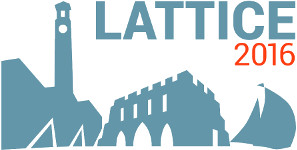#The 34th International Symposium on Lattice Field Theory (Lattice 2016)

Jul 24 – 30, 2016
Highfield Campus, University of Southampton
Europe/London timezone

## An $a_0$ resonance in strongly coupled $\pi \eta$, $K \overline{K}$ scattering from lattice QCD

Jul 26, 2016, 4:50 PM
20m
Building B2a Room 2065 (Highfield Campus, University of Southampton)

### Speaker

Jozef Dudek (Jefferson Lab)

### Description

We present the first calculation of coupled-channel meson-meson scattering in the isospin $=1$, $G$-parity negative sector, with channels $\pi \eta$, $K\overline{K}$ and $\pi \eta'$, in a first-principles approach to QCD. From the discrete spectrum of eigenstates in three volumes extracted from lattice QCD correlation functions we determine the energy dependence of the $S$-matrix, and find that the $S$-wave features a prominent cusp-like structure in $\pi \eta \to \pi \eta$ close to $K\overline{K}$ threshold coupled with a rapid turn on of amplitudes leading to the $K\overline{K}$ final-state. This behavior is traced to an $a_0(980)$-like resonance, strongly coupled to both $\pi \eta$ and $K\overline{K}$, which is identified with a pole in the complex energy plane, appearing on only a single unphysical Riemann sheet. Consideration of $D$-wave scattering suggests a narrow tensor resonance at higher energy.

### Primary author

Jozef Dudek (Jefferson Lab)

 Slides